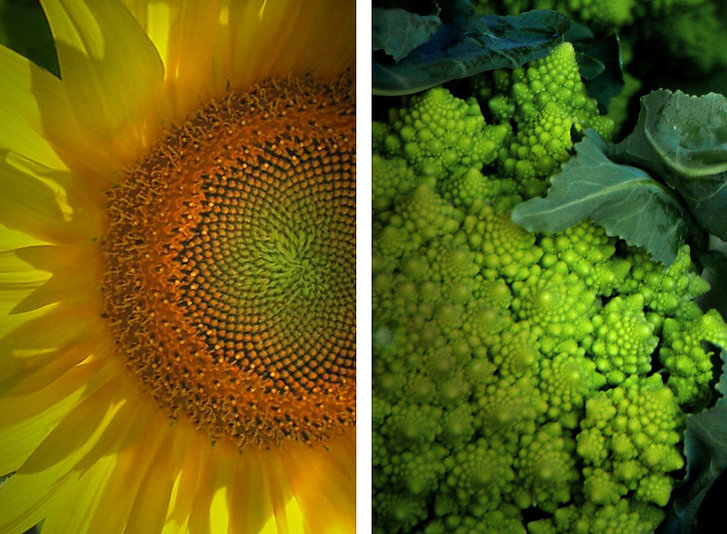# Fibonacci

Aspects of Design

Leonardo Fibonacci was an Italian mathematician (approx. 1170 – 1240). He was the first European mathematician to introduce the numerical sequence, described below, to the western world. The same numerical sequence had been used in ancient India since 200BC (1)###### ​

The Fibonacci numbers are a series of numbers where each number is the sum of the previous two numbers.

Starting with 1 we get:
1+0=1
1+1=2
1+2=3
2+3=5
3+5=8
5+8=13
8+13=21
13+21=34 and so on

The further this sequence goes, the closer the ratio between adjacent numbers gets to the Golden Mean. No matter how far the sequence is expanded it never reaches the 1:1.618 ratio of the Golden Mean

###### Fibonacci SpiralThe Fibonacci sequence can be seen in many natural growth patterns. If we superimpose a grid of rectangles, of Fibonacci proportions, over the exponential spiral of an abalone shell, we will see that it closely matches the natural growth spiral.

Similar patterns can be found in the growth spirals found in many plants. The number of clockwise spirals on the face of a sunflower will always be a Fibonacci number. The number of anti-clockwise spirals on the same plant will be a consecutive Fibonacci number. The largest such numbers recorded are 144 and 233 (2)The bidirectional spirals on sunflower heads and Romanesco Broccoli will be consecutive Fibonacci numbers in the clockwise and anti clockwise direction.

Like the Golden mean, the Fibonacci sequence is mathematically fascinating but aesthetically, its complexities are of minor practical importance.